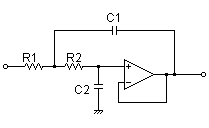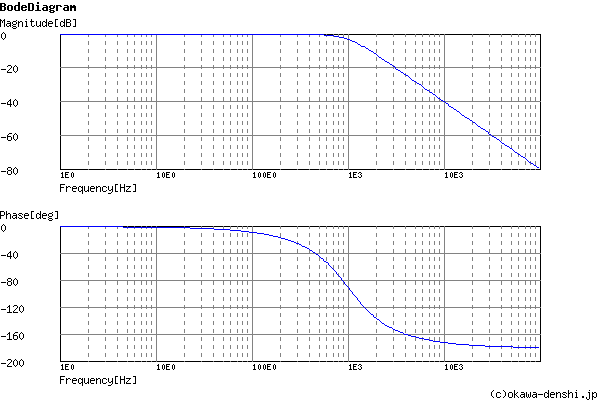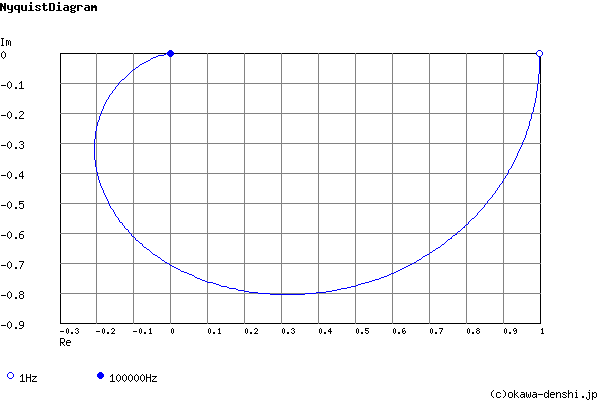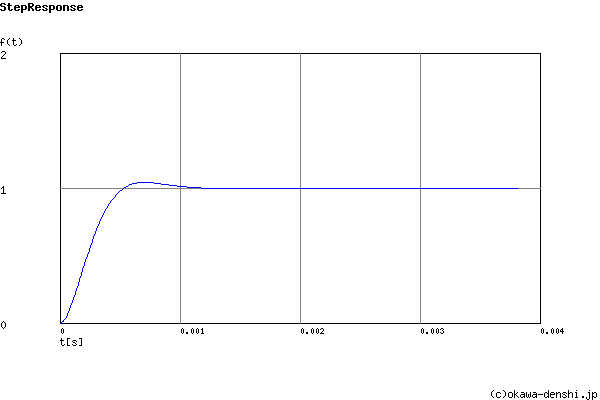# (Sample)Sallen-Key Low-pass Filter Design Tool - Result -

Calculated the Transfer Function for the Sallen-Key low-pass filter, displayed on graphs, showing Bode diagram, Nyquist diagram, Impulse response and Step response.

## Sallen-Key Low-pass Filter

 Vin(s)→→Vout(s)
(Sample)Transfer Function:
 G(s)= 39401103.2309 s2+8888.88888889s+39401103.2309

R1 = 30kΩ
R2 = 18kΩ
C1 = 0.01uF
C2 = 0.0047uF

#### Cut-off frequency

fc = 999.020322137[Hz]

#### Quality factor

Q = 0.706165853583

#### Damping ratio

ζ = 0.708048962525

#### Pole(s)

p = -707.355302631 +705.471530171i[Hz]
|p|= 999.020322137[Hz]
p = -707.355302631-705.471530171i[Hz]
|p|= 999.020322137[Hz]

#### Phase margin

pm= NAN[deg] (f =0[Hz])

#### Oscillation frequency

f = 705.471530171[Hz]

#### Overshoot (in absolute value)

The 1st peak  gpk = 1.04 (t =0.00071[sec])
The 2nd peak  gpk = 1 (t =0.0014[sec])
The 3rd peak  gpk = 1 (t =0.0021[sec])

g(∞) = 1

fc=Hz

### Q factor | Damping ratio ζ

Quality factor Q =
Damping ratio ζ =
 C1 = F C2 = F
C1, C2 is optional. But when setting these capacitances, C1 and C2 of both are needed to give following the equation
(C2/C1) ≤ ζ 2
(C1/C2) ≥ 4Q2

Select Capacitor Sequence:
Select Resistor Sequence:

### Frequency analysis

Bode diagram
Phase  Group delay
Nyquist diagram
Pole, zero
Phase margin
Oscillation analysis
Analysis on frequency range:
f1=∼f2=[Hz] (optional)

### Transient analysis

Step response
Impulse response
Overshoot
Final value of the step response
Analysis on time range:
0∼[sec] (optional)

# Frequency analysis# Transient analysis